Tutorials
python

# Python Sets and Set Theory

Learn about Python sets: what they are, how to create them, when to use them, built-in functions, and their relationship to set theory operations.

## Sets vs Lists and Tuples

Lists and tuples are standard Python data types that store values in a sequence. Sets are another standard Python data type that also store values. The major difference is that sets, unlike lists or tuples, cannot have multiple occurrences of the same element and store unordered values.

## Advantages of Python Sets

Because sets cannot have multiple occurrences of the same element, it makes sets highly useful to efficiently remove duplicate values from a list or tuple and to perform common math operations like unions and intersections.

If you'd like to sharpen your Python skills, or you're just a beginner, be sure to take a look at our Python Programmer career track on DataCamp.

This tutorial will introduce you a few topics about Python sets and set theory:

With that, let's get started.

## Initialize a Set

Sets are a mutable collection of distinct (unique) immutable values that are unordered.

You can initialize an empty set by using set().

emptySet = set()


To intialize a set with values, you can pass in a list to set().

dataScientist = set(['Python', 'R', 'SQL', 'Git', 'Tableau', 'SAS'])
dataEngineer = set(['Python', 'Java', 'Scala', 'Git', 'SQL', 'Hadoop'])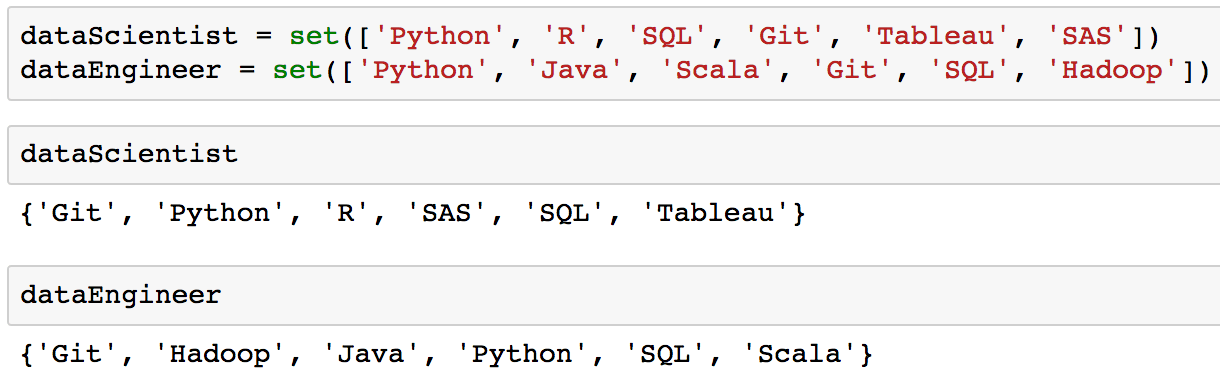If you look at the output of dataScientist and dataEngineer variables above, notice that the values in the set are not in the order added in. This is because sets are unordered.

Sets containing values can also be initialized by using curly braces.

dataScientist = {'Python', 'R', 'SQL', 'Git', 'Tableau', 'SAS'}
dataEngineer = {'Python', 'Java', 'Scala', 'Git', 'SQL', 'Hadoop'}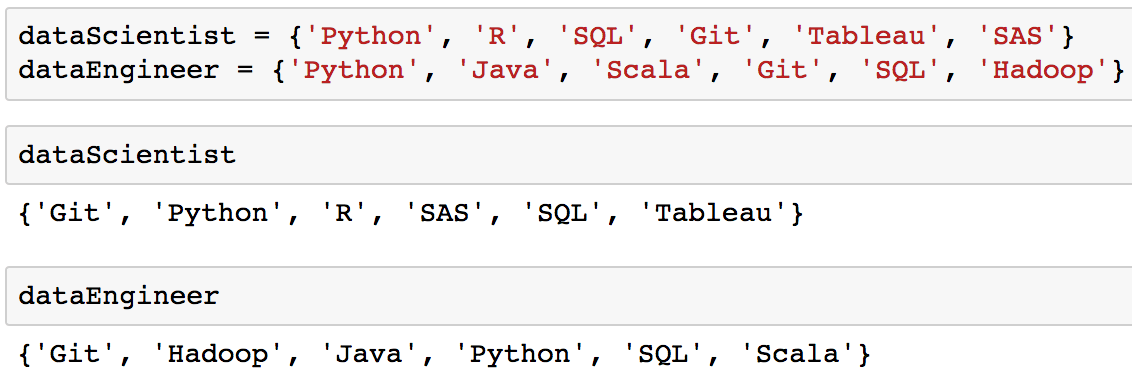Keep in mind that curly braces can only be used to initialize a set containing values. The image below shows that using curly braces without values is one of the ways to initialize a dictionary and not a set.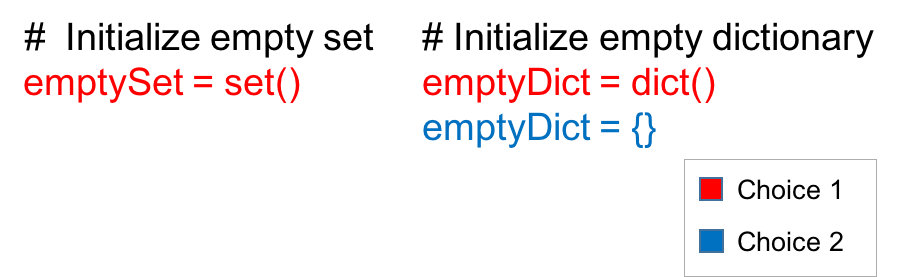## Add and Remove Values from Sets

To add or remove values from a set, you first have to initialize a set.

# Initialize set with values
graphicDesigner = {'InDesign', 'Photoshop', 'Acrobat', 'Premiere', 'Bridge'}


### Add Values to a Set

You can use the method add to add a value to a set.

graphicDesigner.add('Illustrator')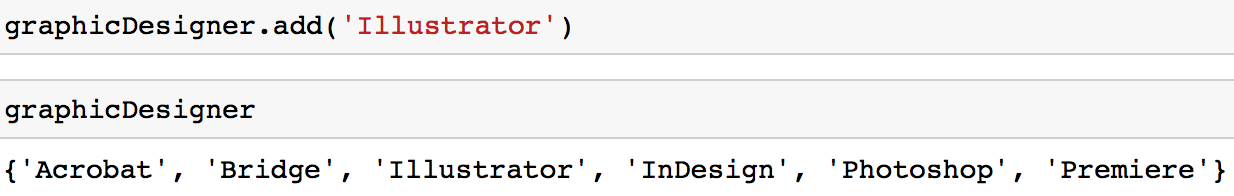In is important to note that you can only add a value that is immutable (like a string or a tuple) to a set. For example, you would get a TypeError if you try to add a list to a set.

graphicDesigner.add(['Powerpoint', 'Blender'])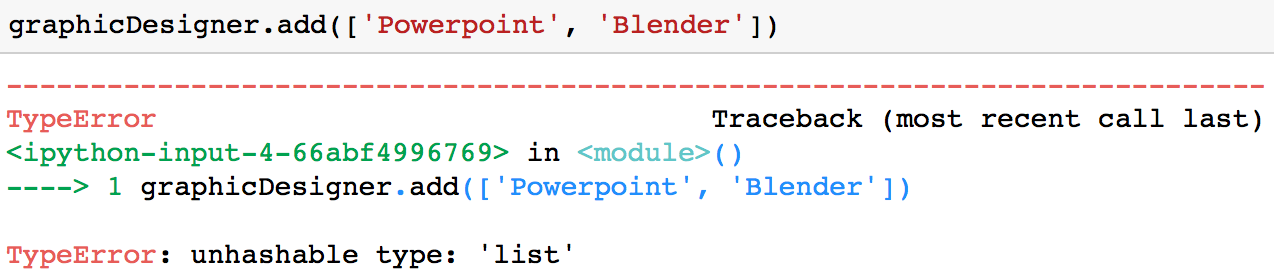## Remove Values from a Set

There are a couple ways to remove a value from a set.

Option 1: You can use the remove method to remove a value from a set.

graphicDesigner.remove('Illustrator')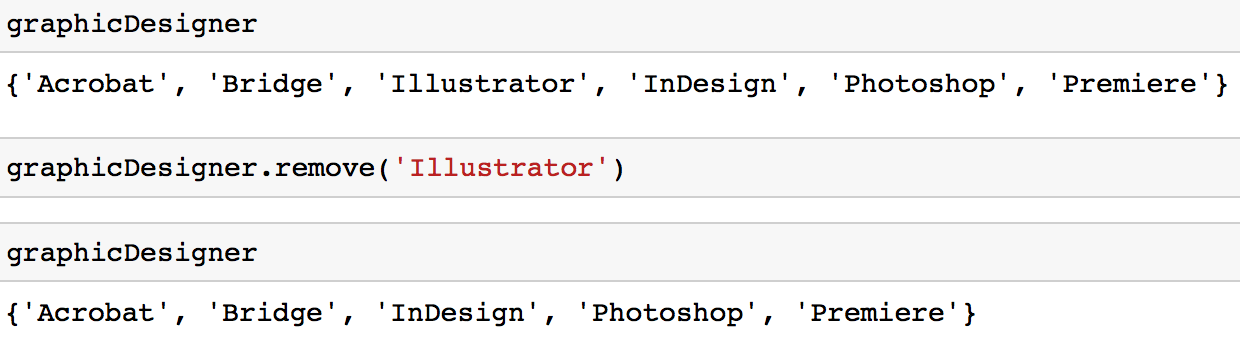The drawback of this method is that if you try to remove a value that is not in your set, you will get a KeyError.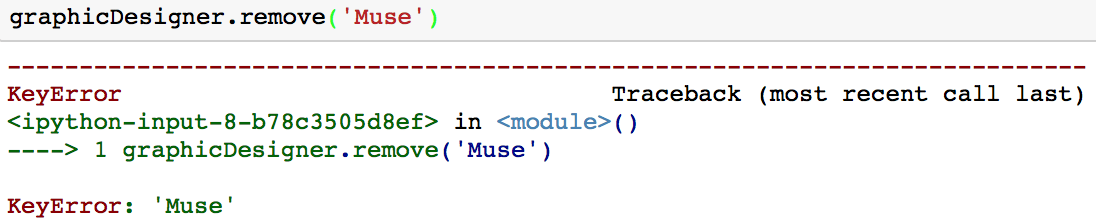Option 2: You can use the discard method to remove a value from a set.

graphicDesigner.discard('Premiere')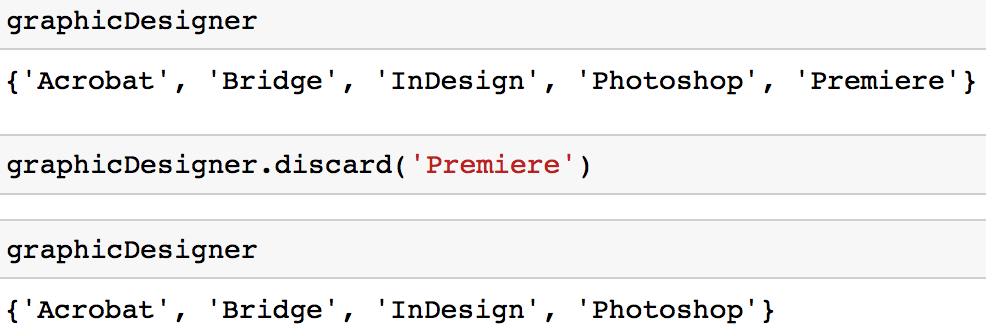The benefit of this approach over the remove method is if you try to remove a value that is not part of the set, you will not get a KeyError. If you are familiar with dictionaries, you might find that this works similarly to the dictionary method get.

Option 3: You can also use the pop method to remove and return an arbitrary value from a set.

graphicDesigner.pop()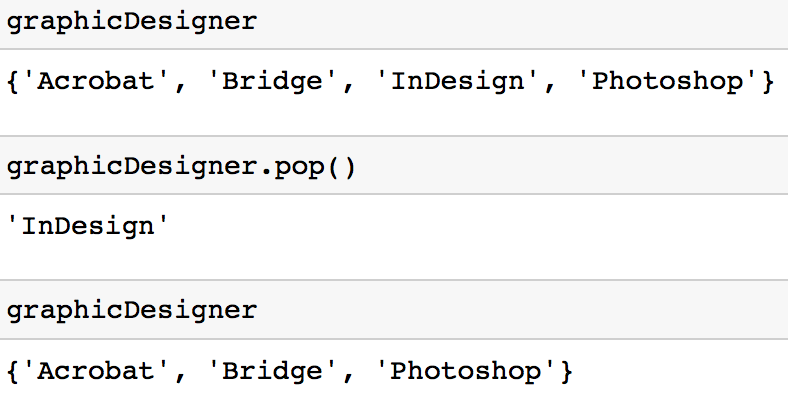It is important to note that the method raises a KeyError if the set is empty.

## Remove All Values from a Set

You can use the clear method to remove all values from a set.

graphicDesigner.clear()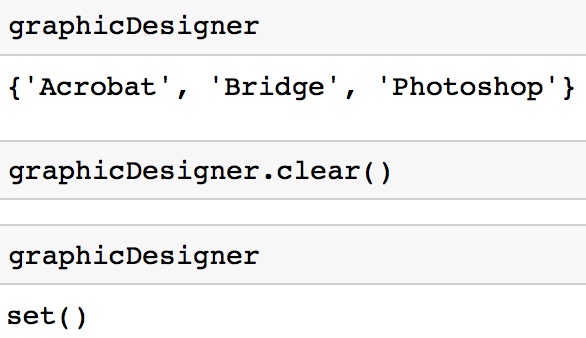## Iterate through a Set

Like many standard python data types, it is possible to iterate through a set.

# Initialize a set
dataScientist = {'Python', 'R', 'SQL', 'Git', 'Tableau', 'SAS'}

for skill in dataScientist:
print(skill)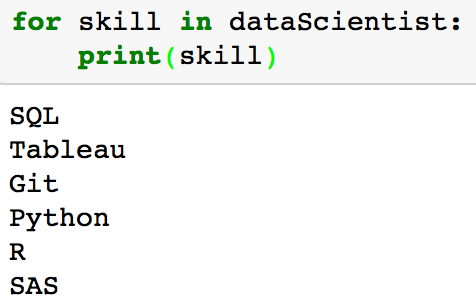If you look at the output of printing each of the values in dataScientist, notice that the values printed in the set are not in the order they were added in. This is because sets are unordered.

## Transform Set into Ordered Values

This tutorial has emphasized that sets are unordered. If you find that you need to get the values from your set in an ordered form, you can use the sorted function which outputs a list that is ordered.

type(sorted(dataScientist))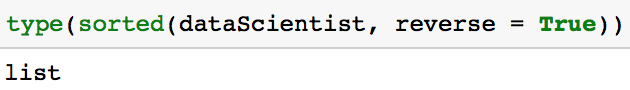The code below outputs the values in the set dataScientist in descending alphabetical order (Z-A in this case).

sorted(dataScientist, reverse = True)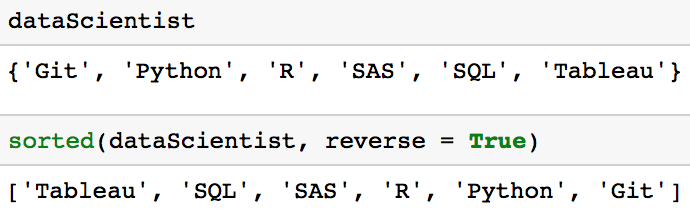## Remove Duplicates from a List

Part of the content in this section was previously explored in the tutorial 18 Most Common Python List Questions, but it is important to emphasize that sets are the fastest way to remove duplicates from a list. To show this, let's study the performance difference between two approaches.

Approach 1: Use a set to remove duplicates from a list.

print(list(set([1, 2, 3, 1, 7])))


Approach 2: Use a list comprehension to remove duplicates from a list (If you would like a refresher on list comprehensions, see this tutorial).

def remove_duplicates(original):
unique = []
[unique.append(n) for n in original if n not in unique]
return(unique)

print(remove_duplicates([1, 2, 3, 1, 7]))


The performance difference can be measured using the the timeit library which allows you to time your Python code. The code below runs the code for each approach 10000 times and outputs the overall time it took in seconds.

import timeit

# Approach 1: Execution time
print(timeit.timeit('list(set([1, 2, 3, 1, 7]))', number=10000))

# Approach 2: Execution time
print(timeit.timeit('remove_duplicates([1, 2, 3, 1, 7])', globals=globals(), number=10000))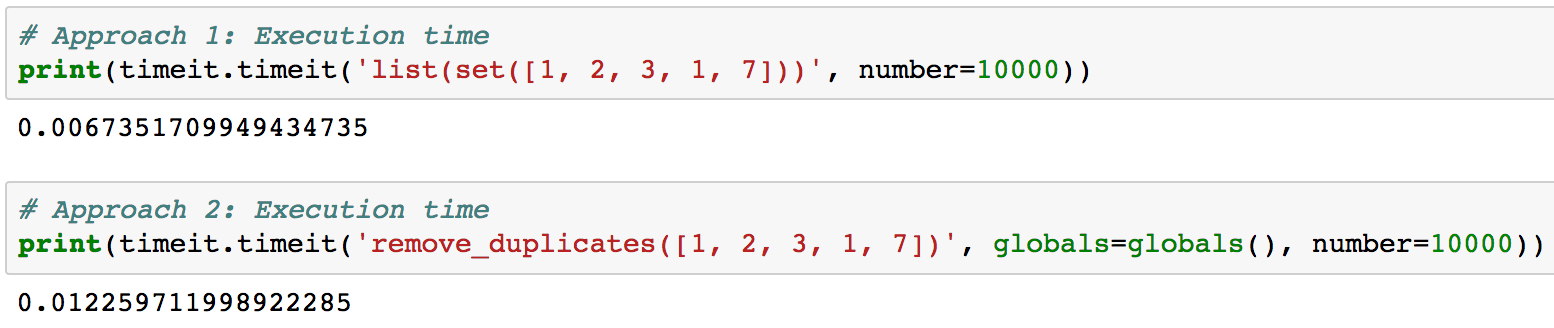Comparing these two approaches shows that using sets to remove duplicates is more efficient. While it may seem like a small difference in time, it can save you a lot of time if you have very large lists.

## Set Operation Methods

A common use of sets in Python is computing standard math operations such as union, intersection, difference, and symmetric difference. The image below shows a couple standard math operations on two sets A and B. The red part of each Venn diagram is the resulting set of a given set operation.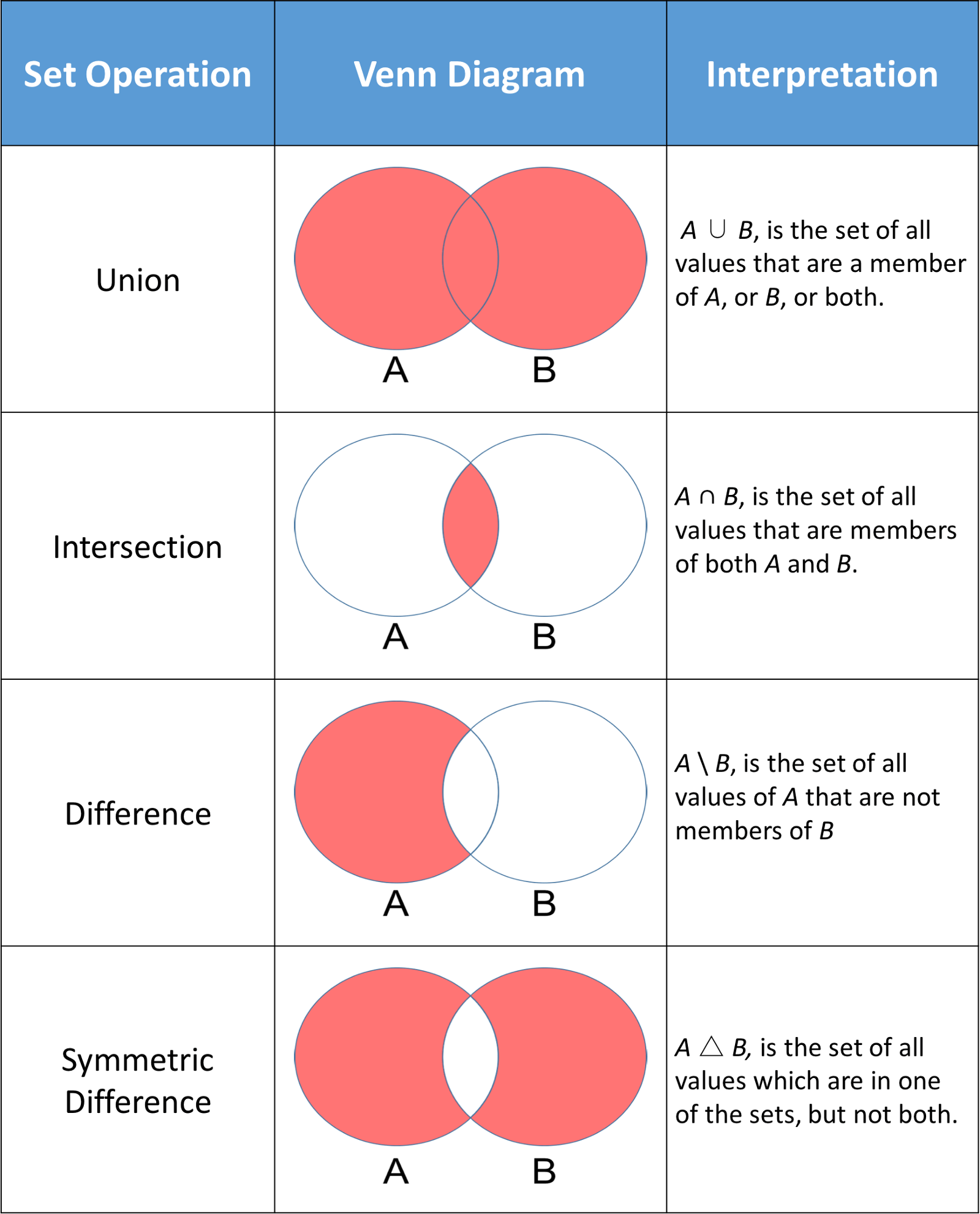Python sets have methods that allow you to perform these mathematical operations as well as operators that give you equivalent results.

Before exploring these methods, let's start by initializing two sets dataScientist and dataEngineer.

dataScientist = set(['Python', 'R', 'SQL', 'Git', 'Tableau', 'SAS'])
dataEngineer = set(['Python', 'Java', 'Scala', 'Git', 'SQL', 'Hadoop'])


### union

A union, denoted dataScientist ∪ dataEngineer, is the set of all values that are values of dataScientist, or dataEngineer, or both. You can use the union method to find out all the unique values in two sets.

# set built-in function union
dataScientist.union(dataEngineer)

# Equivalent Result
dataScientist | dataEngineer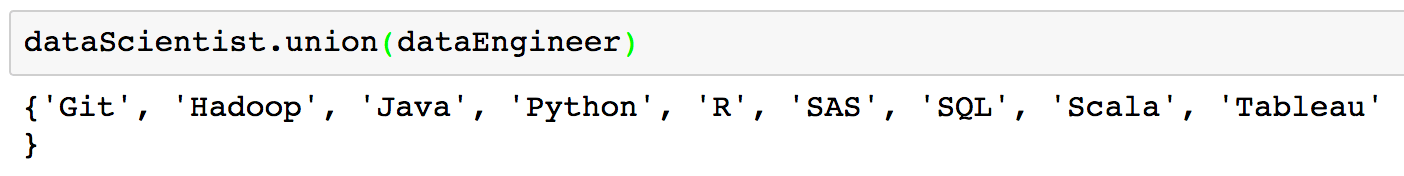The set returned from the union can be visualized as the red part of the Venn diagram below.### intersection

An intersection of two sets dataScientist and dataEngineer, denoted dataScientist ∩ dataEngineer, is the set of all values that are values of both dataScientist and dataEngineer.

# Intersection operation
dataScientist.intersection(dataEngineer)

# Equivalent Result
dataScientist & dataEngineer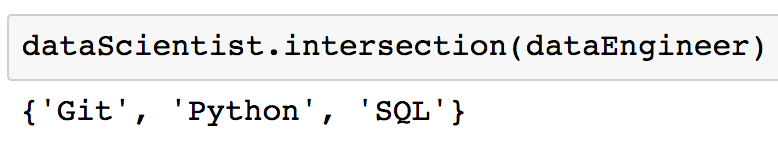The set returned from the intersection can be visualized as the red part of the Venn diagram below.You may find that you come across a case where you want to make sure that two sets have no value in common. In order words, you want two sets that have an intersection that is empty. These two sets are called disjoint sets. You can test for disjoint sets by using the isdisjoint method.

# Initialize a set
graphicDesigner = {'Illustrator', 'InDesign', 'Photoshop'}

# These sets have elements in common so it would return False
dataScientist.isdisjoint(dataEngineer)

# These sets have no elements in common so it would return True
dataScientist.isdisjoint(graphicDesigner)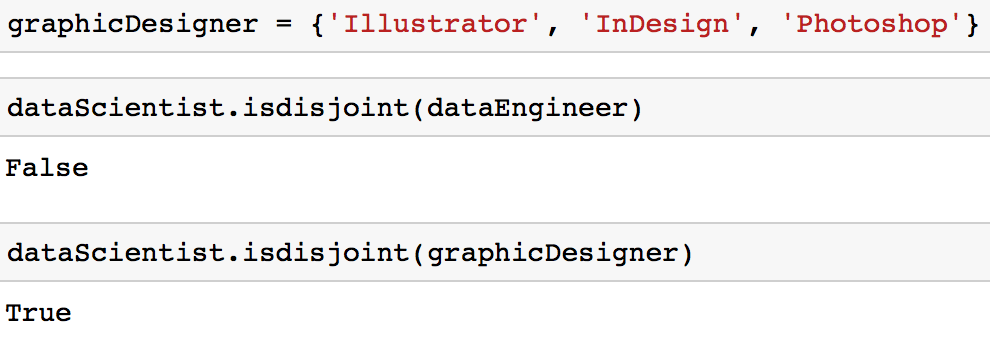You can notice in the intersection shown in the Venn diagram below that the disjoint sets dataScientist and graphicDesigner have no values in common.## difference

A difference of two sets dataScientist and dataEngineer, denoted dataScientist \ dataEngineer, is the set of all values of dataScientist that are not values of dataEngineer.

# Difference Operation
dataScientist.difference(dataEngineer)

# Equivalent Result
dataScientist - dataEngineer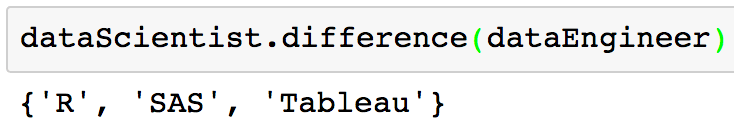The set returned from the difference can be visualized as the red part of the Venn diagram below.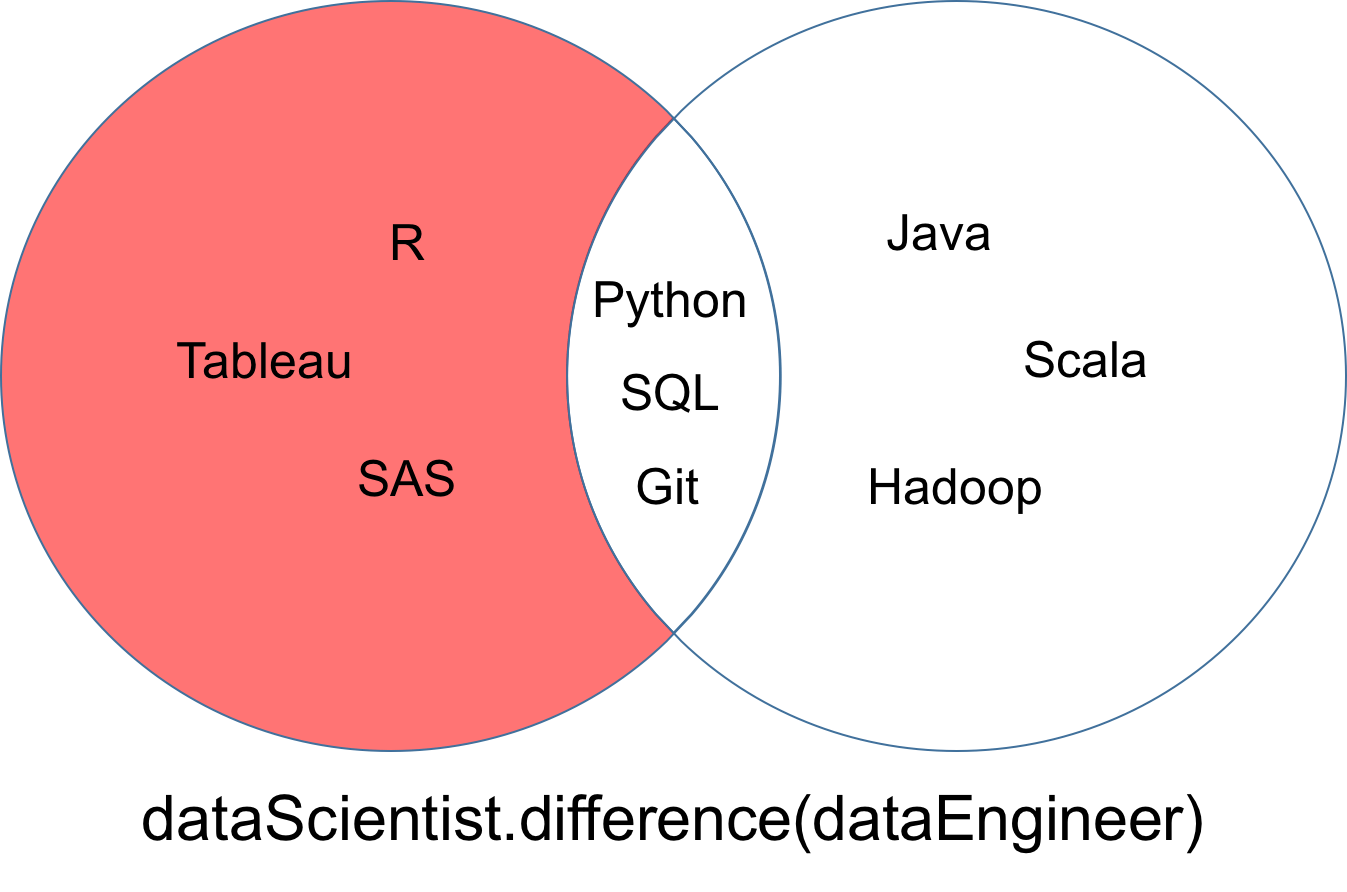## symmetric_difference

A symmetric difference of two sets dataScientist and dataEngineer, denoted dataScientist △ dataEngineer, is the set of all values that are values of exactly one of two sets, but not both.

# Symmetric Difference Operation
dataScientist.symmetric_difference(dataEngineer)

# Equivalent Result
dataScientist ^ dataEngineer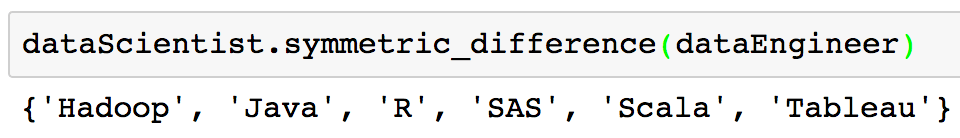The set returned from the symmetric difference can be visualized as the red part of the Venn diagram below.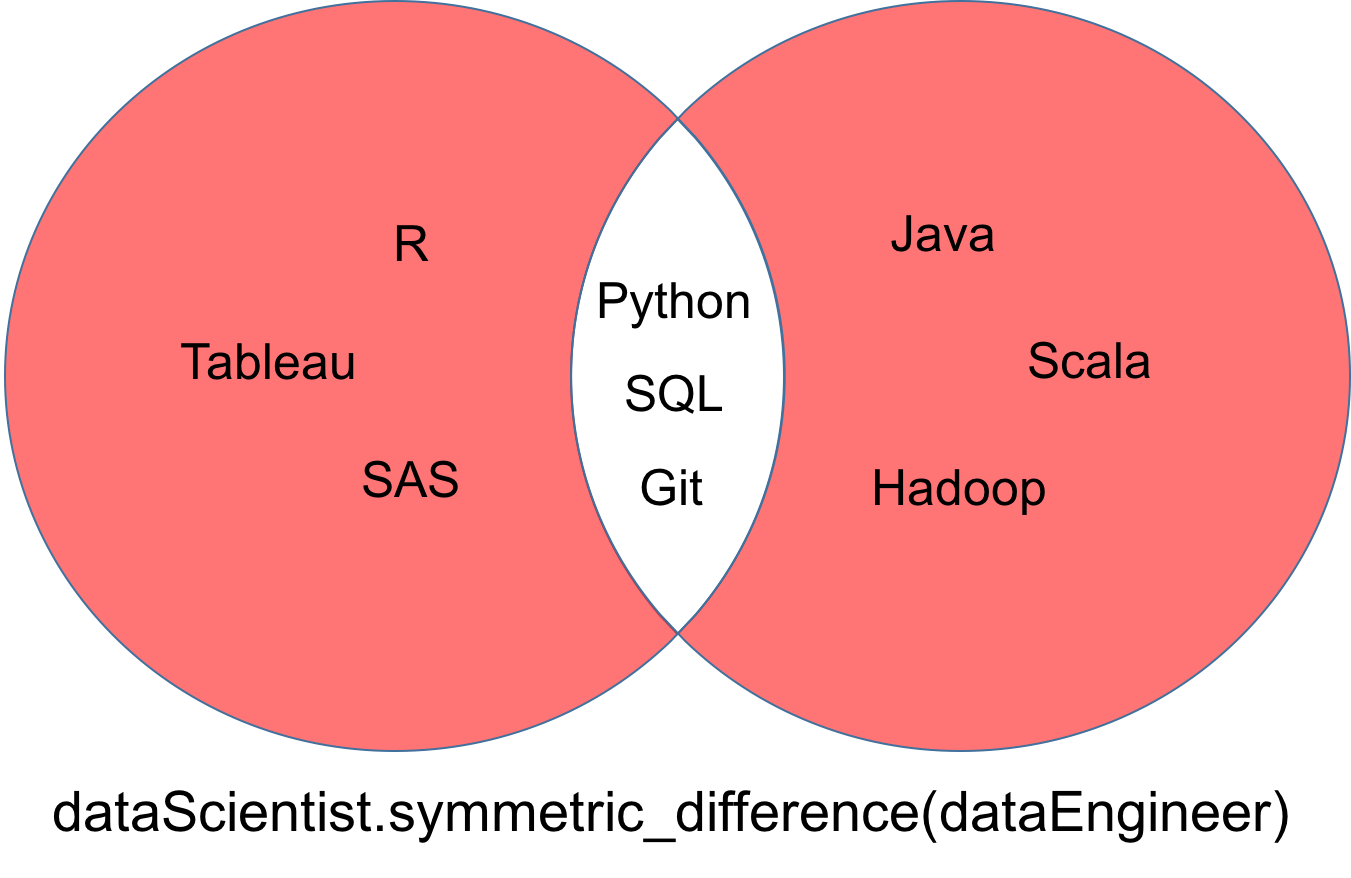## Set Comprehension

You may have previously have learned about list comprehensions, dictionary comprehensions, and generator comprehensions. There is also set comprehensions. Set comprehensions are very similar. Set comprehensions in Python can be constructed as follows:

{skill for skill in ['SQL', 'SQL', 'PYTHON', 'PYTHON']}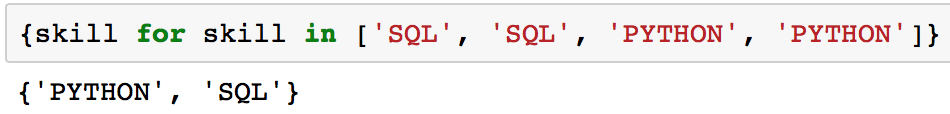The output above is a set of 2 values because sets cannot have multiple occurences of the same element.

The idea behind using set comprehensions is to let you write and reason in code the same way you would do mathematics by hand.

{skill for skill in ['GIT', 'PYTHON', 'SQL'] if skill not in {'GIT', 'PYTHON', 'JAVA'}}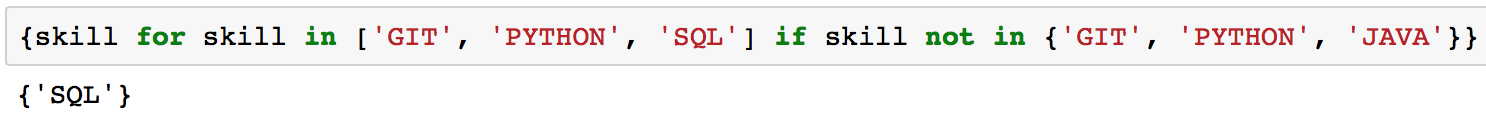The code above is similar to a set difference you learned about earlier. It just looks a bit different.

## Membership Tests

Membership tests check whether a specific element is contained in a sequence, such as strings, lists, tuples, or sets. One of the main advantages of using sets in Python is that they are highly optimized for membership tests. For example, sets do membership tests a lot more efficiently than lists. In case you are from a computer science background, this is because the average case time complexity of membership tests in sets are O(1) vs O(n) for lists.

The code below shows a membership test using a list.

# Initialize a list
possibleList = ['Python', 'R', 'SQL', 'Git', 'Tableau', 'SAS', 'Java', 'Spark', 'Scala']

# Membership test
'Python' in possibleList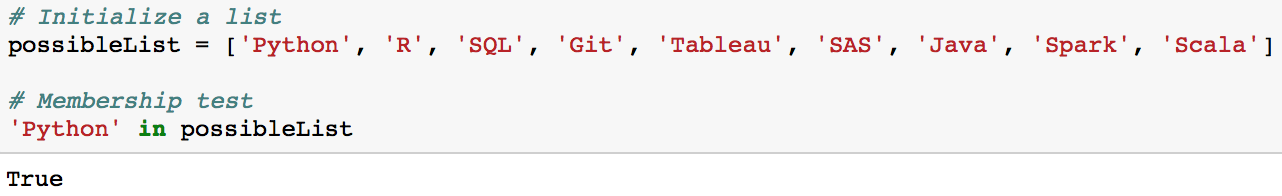Something similar can be done for sets. Sets just happen to be more efficient.

# Initialize a set
possibleSet = {'Python', 'R', 'SQL', 'Git', 'Tableau', 'SAS', 'Java', 'Spark', 'Scala'}

# Membership test
'Python' in possibleSet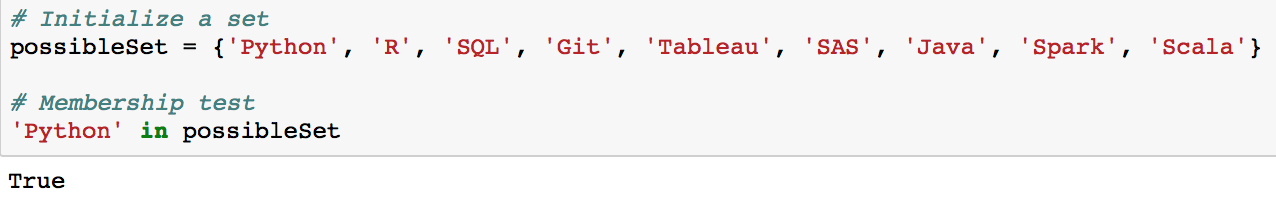Since possibleSet is a set and the value 'Python' is a value of possibleSet, this can be denoted as 'Python'possibleSet.

If you had a value that wasn't part of the set, like 'Fortran', it would be denoted as 'Fortran'possibleSet.

## Subset

A practical application of understanding membership is subsets.

Let's first initialize two sets.

possibleSkills = {'Python', 'R', 'SQL', 'Git', 'Tableau', 'SAS'}
mySkills = {'Python', 'R'}


If every value of the set mySkills is also a value of the set possibleSkills, then mySkills is said to be a subset of possibleSkills, mathematically written mySkillspossibleSkills.

You can check to see if one set is a subset of another using the method issubset.

mySkills.issubset(possibleSkills)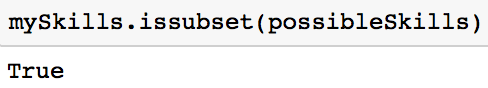Since the method returns True in this case, it is a subset. In the Venn diagram below, notice that every value of the set mySkills is also a value of the set possibleSkills.## Frozensets

You have have encountered nested lists and tuples.

# Nested Lists and Tuples
nestedLists = [['the', 12], ['to', 11], ['of', 9], ['and', 7], ['that', 6]]
nestedTuples = (('the', 12), ('to', 11), ('of', 9), ('and', 7), ('that', 6))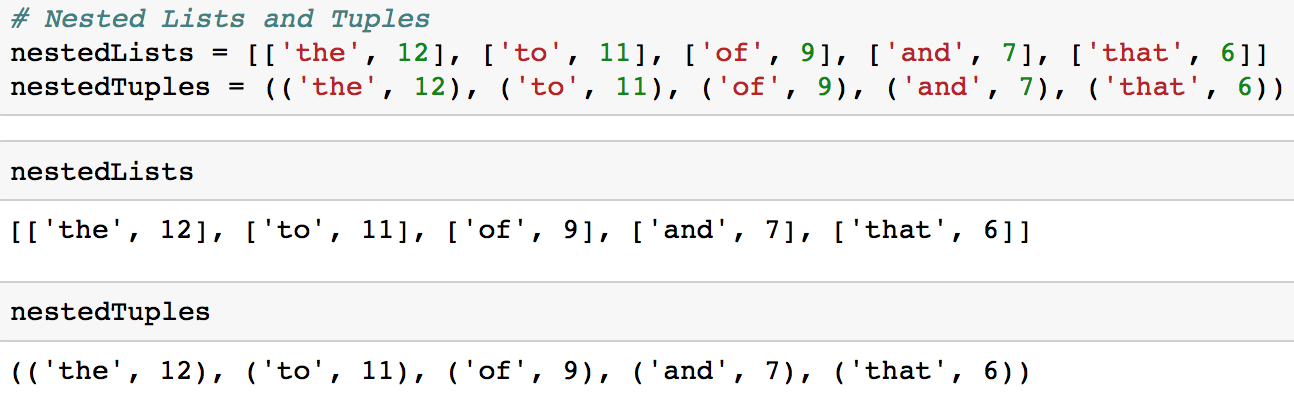The problem with nested sets is that you cannot normally have nested sets as sets cannot contain mutable values including sets.This is one situation where you may wish to use a frozenset. A frozenset is very similar to a set except that a frozenset is immutable.

You make a frozenset by using frozenset().

# Initialize a frozenset
immutableSet = frozenset()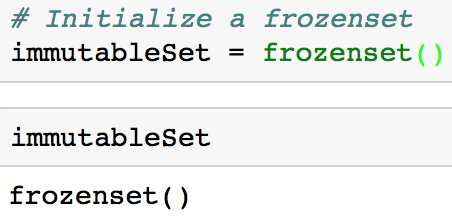You can make a nested set if you utilize a frozenset similar to the code below.

nestedSets = set([frozenset()])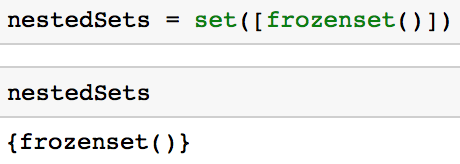It is important to keep in mind that a major disadvantage of a frozenset is that since they are immutable, it means that you cannot add or remove values.

# Conclusion

The Python sets are highly useful to efficiently remove duplicate values from a collection like a list and to perform common math operations like unions and intersections. Some of the challenges people often encounter are when to use the various data types. For example, if you feel like you aren't sure when it is advantageous to use a dictionary versus a set, I encourage you to check out DataCamp's daily practice mode. If you any questions or thoughts on the tutorial, feel free to reach out in the comments below or through Twitter.

Want to leave a comment?